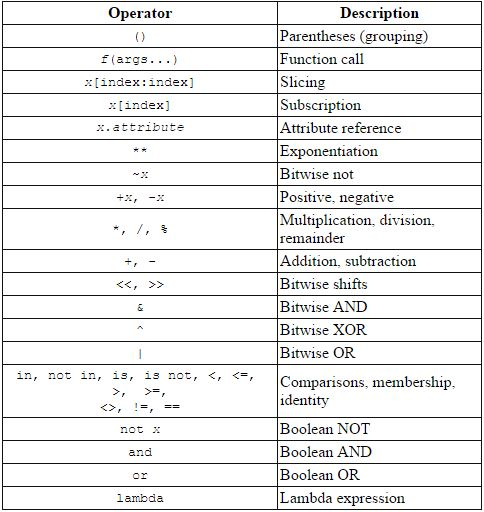# Python Numbers

Updated on May 07, 2020

This data type supports only numerical values like 1, 31.4, -1000, 0.000023, 88888888.

Python supports 3 different numerical types.

1. int - for integer values like 1, 100, 2255, -999999, 0, 12345678.
2. float - for floating-point values like 2.3, 3.14, 2.71, -11.0.
3. complex - for complex numbers like 3+2j, -2+2.3j, 10j, 4.5+3.14j.

## Integers #

Integer literals in python belong to int class.

 1 2 3 >>> i = 100 >>> i 100 

## Floats #

Floating points are values with decimal point like.

 1 2 3 >>> f = 12.3 >>> f 12.3 

One point to note that when one of the operands for numeric operators is a float value then the result will be in float value.

 1 2 >>> 3 * 1.5 4.5 

## Complex number #

As you may know complex number consists of two parts real and imaginary, and is denoted by j. You can define complex number like this:

>>> x = 2 + 3j # where 2 is the real part and 3 is imaginary


## Determining types #

Python has type() inbuilt function which is use to determine the type of the variable.

 1 2 3 >>> x = 12 >>> type(x)   

## Python operators #

Python has the different operators which allows you to carry out required calculations in your program.

+  , -  and * works as expected, remaining operators require some explanation.

Name Meaning Example Rresult
+ Addition 15+20 35
- Subtraction 24.5-3.5 21.0
* Multiplication 15*4 60
/ Float Division 4/5 0.8
// Integer Division 4//5 0
** Exponentiation 4**2 16
% Remainder 27%4 3

Float Division (/) : The /  operator divides and return result as floating point number means it will always return fractional part. For e.g

 1 2 >>> 3/2  1.5 

Integer Division (//) : The // perform integer division i.e it will truncate the decimal part of the answer and return only integer.

 1 2 >>> 3//2  1 

Exponentiation Operator (**) : This operator helps to compute ab (a raise to the power of b). Let's take an example:

>>> 2 ** 3 # is same as 2 * 2 * 2
8

Remainder operator (%) : The % operator also known as remainder or modulus operator. This operator return remainder after division. For e.g:

 1 2 >>> 7 % 2 1 

## Operator Precedence #

In python every expression are evaluated using operator precedence. Let's take an example to make it clear.

>>> 3 * 4 + 1


In the above expression which operation will be evaluated first addition or multiplication? To answer such question we need to refer to operator precedence list in python. Image below list python precedence order from high to low.As you can see in table above * is above +, so * will occur first then addition. Therefore the result of the above expression will be 13.

 1 2 >>> 3 * 4 + 1 >>> 13 

Let's,take one more example to illustrate one more concept.

>>> 3 + 4 - 2


In above expression which will occur first addition or subtraction. As we can see from the table + and - have same precedence, then they will be evaluated from left to right, i.e addition will be applied first then subtraction.

 1 2 >>> 3 + 4 - 2 >>> 5 

The only exception to this rule is assignment operator (=) which occur from right to left.

a = b = c


You can change precedence by using parentheses (),  For e.g:

 1 2 >> 3 * (4 + 1) >> 15 

As you can see from the precedence table () has highest priority so in expression 3 * (4 + 1), (4 + 1) is evaluated first then multiplication. Hence you can use () to alter order of precedence.

## Augmented Assignment Operator #

These operator allows you write shortcut assignment statements. For e.g:

 1 2 3 4 >>> count = 1 >>> count = count + 1 >>> count 2 

by using Augmented Assignment Operator we can write it as:

 1 2 3 4 >>> count = 1 >>> count += 1 >>> count 2 

Similarly you can use -, %, //, /, *, **  with assignment operator to form augmented assignment operator.

Operator Name Example Equivalent
+= Addition assignment x += 4 x = x + 4
-= Subtraction assignment x -= 2 x = x - 2
*= Multiplication assignment x *= 5 x = x * 5
/*= Division assignment x /= 5 x = x / 5
//*= Integer division assignment x //= 5 x = x // 5
%*= Remainder assignment x %= 5 x = x % 5
**= Exponent assignment x **= 5 x = x ** 5

In the next post we will learn about python strings.